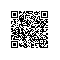# PyODPS DataFrame 处理笛卡尔积的几种方式

PyODPS 提供了 DataFrame API 来用类似 pandas 的接口进行大规模数据分析以及预处理，本文主要介绍如何使用 PyODPS 执行笛卡尔积的操作。

## haversine 公式

def  haversine(lat1,  lon1,  lat2,  lon2):
#  lat1,  lon1  为位置  1  的经纬度坐标
#  lat2,  lon2  为位置  2  的经纬度坐标
import  numpy  as  np

a  =  np.sin(  dlat  /2  )  **2  +  np.cos(np.radians(lat1))  *  np.cos(np.radians(lat2))  *  np.sin(  dlon  /2  )  **2
c  =  2  *  np.arcsin(np.sqrt(a))
r  =  6371  #  地球平均半径，单位为公里
return  c  *  r

## MapJoin

In  :  df1  =  o.get_table('coordinates1').to_df()

In  :  df2  =  o.get_table('coordinates2').to_df()

In  :  df3  =  df1.join(df2,  mapjoin=True)

In  :  df1.schema
Out:
odps.Schema  {
latitude                    float64
longitude                  float64
id                                string
}

In  :  df2.schema
Out:
odps.Schema  {
latitude                    float64
longitude                  float64
id                                string
}

In  :  df3.schema
Out:
odps.Schema  {
latitude_x                        float64
longitude_x                      float64
id_x                                    string
latitude_y                        float64
longitude_y                      float64
id_y                                    string
}


In  :  r  =  6371
...:  dis1  =  (df3.latitude_y  -  df3.latitude_x).radians()
...:  dis2  =  (df3.longitude_y  -  df3.longitude_x).radians()
...:  a  =  (dis1  /  2).sin()  **  2  +  df3.latitude_x.radians().cos()  *  df3.latitude_y.radians().cos()  *  (dis2  /  2).sin()  **  2
...:  df3['dis']  =  2  *  a.sqrt().arcsin()  *  r

Out:
latitude_x  longitude_x id_x  latitude_y   longitude_y id_y       dis
0   76.252432    59.628253    0   84.045210     6.517522    0  1246.864981
1   76.252432    59.628253    0   59.061796     0.794939    1  2925.953147
2   76.252432    59.628253    0   42.368304    30.119837    2  4020.604942
3   76.252432    59.628253    0   81.290936    51.682749    3   584.779748
4   76.252432    59.628253    0   34.665222   147.167070    4  6213.944942
5   76.252432    59.628253    0   58.058854   165.471565    5  4205.219179
6   76.252432    59.628253    0   79.150677    58.661890    6   323.070785
7   76.252432    59.628253    0   72.622352   123.195778    7  1839.380760
8   76.252432    59.628253    0   80.063614   138.845193    8  1703.782421
9   76.252432    59.628253    0   36.231584    90.774527    9  4717.284949

In : df1.count()
Out: 2000

In : df2.count()
Out: 100

In : df3.count()
Out: 200000

df3 已经是有 M * N 条数据了，接下来如果需要知道最小距离，直接对 df3 调用 groupby 接上 min 聚合函数就可以得到每个目标点的最小距离。


Out:
dis_min
0   323.070785
1    64.755493
2  1249.283169
3   309.818288
4  1790.484748
5   385.107739
6   498.816157
7   615.987467
8   437.765432
9   272.589621


## DataFrame 自定义函数

### 表资源

## use dataframe udf

df1 = o.get_table('coordinates1').to_df()
df2 = o.get_table('coordinates2').to_df()

def func(collections):
import pandas as pd

collection = collections

ids = []
latitudes = []
longitudes = []
for r in collection:
ids.append(r.id)
latitudes.append(r.latitude)
longitudes.append(r.longitude)

df = pd.DataFrame({'id': ids, 'latitude':latitudes, 'longitude':longitudes})
def h(x):
df['dis'] = haversine(x.latitude, x.longitude, df.latitude, df.longitude)
return df.iloc[df['dis'].idxmin()]['id']
return h

df1[df1.id, df1.apply(func, resources=[df2], axis=1, reduce=True, types='string').rename('min_id')].execute(
libraries=['pandas.zip', 'python-dateutil.zip', 'pytz.zip', 'six.tar.gz'])


### 全局变量

df1 = o.get_table('coordinates1').to_df()
df2 = o.get_table('coordinates2').to_df()
df = df2.to_pandas()

def func(x):
df['dis'] = haversine(x.latitude, x.longitude, df.latitude, df.longitude)
return df.iloc[df['dis'].idxmin()]['id']

df1[df1.id, df1.apply(func, axis=1, reduce=True, types='string').rename('min_id')].execute(
libraries=['pandas.zip', 'python-dateutil.zip', 'pytz.zip', 'six.tar.gz'])

## 总结使用钉钉扫一扫加入圈子
+ 订阅